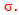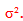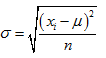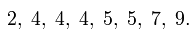Variation

Variation refers to the amount that values vary among themselves or the spread of the data. The three main measures of variation are range, variance, and standard deviation. The range of a data set is the difference between the highest value and the lowest value. Algebraically, the standard deviation measures the spread or average distance each point is from the mean. Graphically, the standard deviation helps to interpret the distribution of a set of numbers. The notation used for standard deviation is the greek letter sigma,The variance describes how far values lie from the mean. The notation used for variance is the greek letter sigma squared,The formula for standard deviation is:Example 1

Find the range of the following set of values:To find the range, subtract the lowest value from the highest value.

9 - 2 = 7

Range = 7